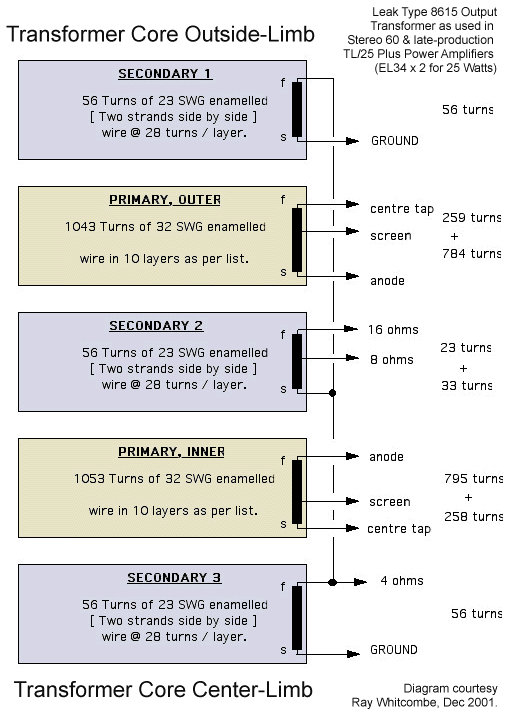Leak 8615 Output Transformer

The Leak 8615 Output transfomer was used in all Stereo 60 power amplifiers, and in late production TL/25 Plus power amplifiers. The 8615 was a revision of the earlier type 3925 transformers used in the Stereo 50 and early production TL/25 Plus power amplifiers.

In December 2001, Ray Whitcombe (who was employed as a Design Engineer at Leak in the 1960's) provided the following details on the winding arrangements inside the 8615 transformer. This data has been obtained by dismantling an 8615 transformer, and would enable any proficient audio transformer company to re-wind a this transformer from new.

Secondary

Top section consists of two layers of 25.5 thou enamelled wire, 23.5 thou with enamel stripped. Each layer consists of 28 turns of two strands of this wire side by side. That is to say 56 turns total.

NB: This arrangement of two wires side by side is used in the 3925 and the 8615 transformer (to lower the leakage inductance by reducing winding height).

Mid section consists of 2 layers of similar wire, but there is a tap ( 8 ohm speaker ) at 33 turns, that is 5 turns into the second layer.

Bottom section is as the top section at 28 + 28 turns in two layers.

This gives total secondary turns as 28+28+28+28+28+28=168 turns in 6 layers.

Primary

Top section ( below top secondary ) consists of 10 layers distributed as follows:

layer 1= 52 turns [ centre tap ]
layer 2= 116 turns
layer 3= 91 turns [ Screen Tap ] 25 turns. ( That is 116 turns tot.)
layer 4= 116 turns
layer 5= 116turns
layer 6= 116 turns
layer 7= 116 turns
layer 8= 116 turns
layer 9= 116 turns
layer 10= 63 turns [ anode ]

That is a total of 1043 turns. The wire is 12.5 thou enamelled or 11 thou stripped. 32 S.W.G.
The lower section of the primary is similar:

layer 1= 52 turns [ anode ]
layer 2= 116 turns
layer 3= 116 turns
layer 4= 116 turns
layer 5= 116turns
layer 6= 116 turns
layer 7= 116 turns
layer 8=47 turns- [[ Screen Tap ] - 62turns. ( That is 109 turns tot.)
layer 9= 116 turns
layer 10= 80 turns [ centre tap ]

That is a total of 1053 turns. The wire is 12.5 thou enamelled or 11 thou stripped. 32 S.W.G

There appears to be a slight inaccuracy in the balance - 1043 versus 1053. This is most likely due to inaccuracies in counting the turns as unwound! Also there will be variations in the original auto-winding.

The transformer laminations are of the E and I type. The centre limb is 1.25 inch wide by 1.75 inch long. The winding space is 5/8 inch. The steel used is 'Grain Oriented' and it is built as a square stack (i.e. 1.25 inch * 1.25 inch).

Primary Impedance

Knowing the total primary and secondary turns, the full-primary impedance is calculated as follows:

Full primary: 1043+1053 = 2096 turns
Full 16-Ohm secondary = 112 turns
Impedance ratio = (2096/112)^2 = 350.2
For 16 Ohm secondary load, primary load anode-to-anode = 16 * 350.2 = 5,604 Ohms

Also the screen taps come in at 25%, as expected.H. J. LEAK & CO. LTD.

Designers and Manufacturers of Specialised Electronic Instruments for the Communications Industries

BRUNEL ROAD * WESTWAY FACTORY ESTATE * LONDON, W3